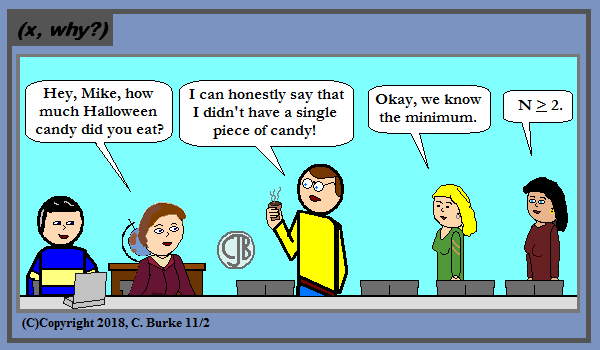## Friday, November 30, 2018

### Random Sketch

(Click on the comic if you can't see the full image.)Emphasis on the 'RANDOM' as it was just one of those things that occurs to me...

Come back often for more funny math and geeky comics.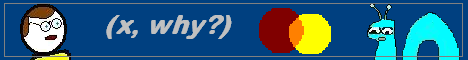## Wednesday, November 28, 2018

### Colors Like My Screen

(Click on the comic if you can't see the full image.)Sometimes I'm like a dog with a pun, er, bone, and I don't want to let it go.

Come back often for more funny math and geeky comics.## Tuesday, November 27, 2018

### Algebra 2 Problems of the Day

Daily Algebra 2 questions and answers.

More Algebra 2 problems.

June 2017, Part II

All Questions in Part II are worth up tp 2 credits. Partial credit is possible.

25. Given r(x) = x3 - 4x2 + 4x - 6, find the value of r(2).

r(2) = (2)3 - 4(2)x2 + 4(2) - 6
r(2) = 8 - 4(4) + 8 - 6 = 8 - 16 + 8 - 6 = -6
Therefore, x - 2 is not a factor of r(x) because r(2) =/= 0. If you divide r(x) / (x - 2) there will be a remainder.

26. The weight of a bag of pears at the local market averages 8 pounds with a standard deviation of 0.5 pound. The weights of all the bags of pears at the market closely follow a normal distribution. Determine what percentage of bags, to the nearest integer, weighed less than 8.25 pounds.

Use your calculator to find a normal distribution. "less than 8.25" means that the endpoints are 0 and 8.25. The mean is 8 and the standard deviation is 0.5.
On your paper, write normalcdf(0,8.25,8,.50) to show work to get full credit. Then enter this into your calculator.
You will get an answer of .6915, which is 69% to the nearest integer.

More Algebra 2 problems.

## Monday, November 26, 2018

### How To Tell the Contradiction

(Click on the comic if you can't see the full image.)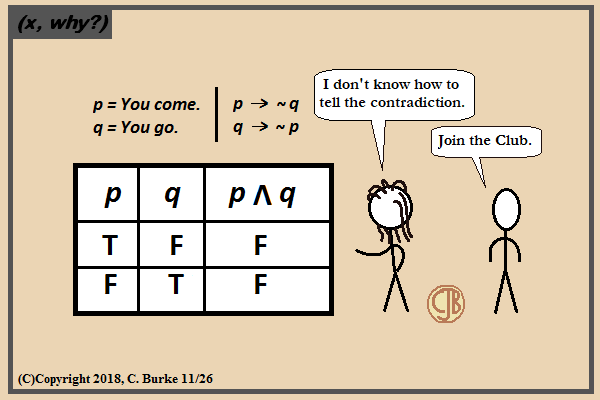Loving would easy if your numbers were like my dreams: fourteen oh three, fourteen oh thre-ee-ee!!

The original truth table for this was going to be way too complicated for this joke.

Oh, and if you're reading this on the blog, this is comic #1403, which just worked out that way. I pretty much just needed a three at the end.

Come back often for more funny math and geeky comics.## Sunday, November 25, 2018

### Algebra 2 Problems of the Day

Daily Algebra 2 questions and answers.

More Algebra 2 problems.

June 2017, Part I

All Questions in Part I are worth 2 credits. No work need be shown. No partial credit.

22. Mallory wants to buy a new window air conditioning unit. The cost for the unit is \$329.99. If she plans to run the unit three months out of the year for an annual operating cost of \$108.78, which function models the cost per year over the lifetime of the unit, C(n), in terms of the number of years, n, that she owns the air conditioner?
(1) C(n) = 329.99 + 108.78n
(2) C(n) = 329.99 + 326.34n
(3) C(n) = (329.99 + 108.78n) / n
(4) C(n) = (329.99 + 326.34n) / n

Answer: (3) C(n) = (329.99 + 108.78n) / n
Choice (1) is the total cost to operate the unit over its lifetime. To find the yearly cost, divide that amount by the number of years, n.
Alternatively, if it costs \$108.78 to operate per year (and then the cost is 108.78n to operate for n years). The cost of the unit is paid once (initial value) and divided by the number of years the unit is owned to get a "per year" cost, which is 329.99/n.
So the yearly cost is: 329.99/n + 108.78, which is equivalent to (329.99 + 108.78n) / n.

23. The expression (-3x2 - 5x + 2) / (x3 + 2x2)

can be rewritten as

As shown in the image below. Factor both the numerator and the denominator.
The numerator is (-1)(3x2 + 5x - 2), which factors into (-1)(3x - 1)(x + 2).
The denominator is (x2)(x + 2).
Eliminate the common factor of x + 2.
At this point, choice (2) looks so close to being correct, but it has a numerator of -3x - 1, instead of -3x + 1.
Use the division rule and reduce the exponents in the numerator by 2, remembering that the last term is actually 1x0.
This gives us choice (4).

24.Jasmine decides to put \$100 in a savings account each month. The account pays 3% annual interest, compounded monthly. How much money, S, will Jasmine have after one year?

Answer: (2) S = (100 - 100(1.0025)12)/(1 - 1.0025)
When compounding interest, if \$100 were deposited initially, with no additional deposits, then choice (3) would have been correct. The interest rat of 3%, or 0.03, is spread out over 12 months. In other words, 0.03/12 = 0.0025 is the monthly interest, raised to the power of 12 for the entire year. You can eliminate choices (1) and (4), both of which use 1.03.
Because additional deposits are being made monthly, choice (3) is incorrect. The more complicated formula shown in choice (2) comes from the Geometric Sequence formula in the reference table at the back of the booklet:

Sn = (a1 - a1rn) / (1 - r), where r =/= 1.
In this case, a1 = 100, and r = 1.0025.

More Algebra 2 problems.

## Thursday, November 22, 2018

### Happy Thanksgiving 2018!

(Click on the comic if you can't see the full image.)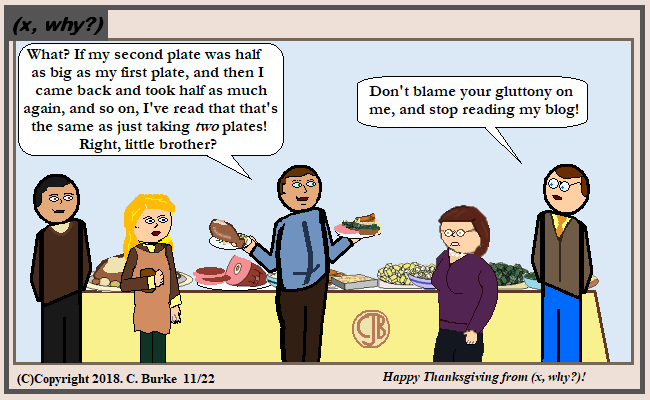Continually taking half as much as the last time is not likely a reasonable assumption. Making an infinite number of trips for more food, on the other hand ...

Happy Thanksgiving, from (x, why?)!

Come back often for more funny math and geeky comics.## Tuesday, November 20, 2018

### Romaine Numerals

(Click on the comic if you can't see the full image.)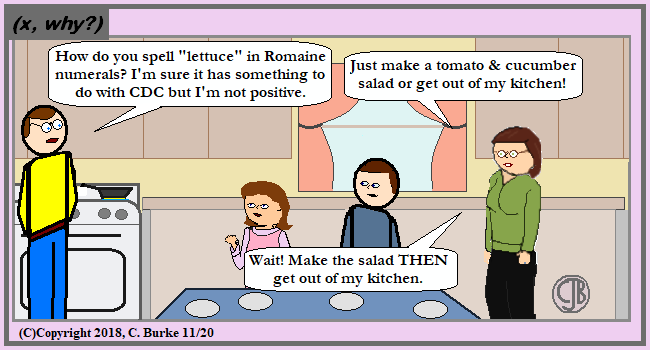Actually, I guess 'CDC' would just be 'D'.

Now "lettuce" dispense with all the other puns, shall we?

Come back often for more funny math and geeky comics.## Friday, November 16, 2018

### Units of Pizza

(Click on the comic if you can't see the full image.)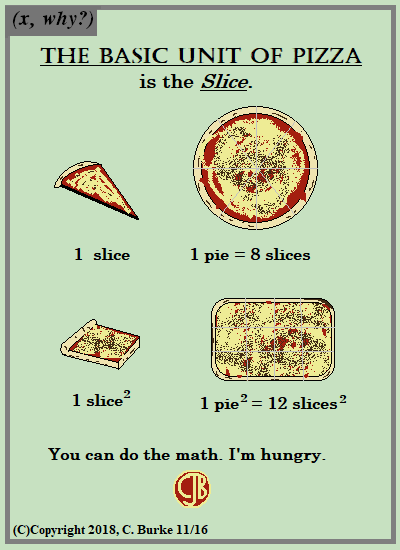Rankings of Best Pizza: Brooklyn; rest of NYC; every place else, does it matter?

Rankings of Best Pizza chains:

Come back often for more funny math and geeky comics.## Wednesday, November 14, 2018

### R. I. P. Stan Lee

(Click on the comic if you can't see the full image.)Excelsior!

I've tried to get away from doing obit comics, but this was one passing that needed to be marked. I knew who Stan Lee was at an early age, even if I didn't read Marvel comics very much. They were a little difficult to get into if you didn't have a good entry point. I prefered the cartoons -- by which I mean "Spider-man! Spider-man! Does what every a spider can!" and "When Captain America throws his mighty shield!"

Yes, I'm old. But you knew that.

It was amazing how quickly the meme pile-up was in this case though.

Come back often for more funny math and geeky comics.## Tuesday, November 13, 2018

### Algebra 2 Problems of the Day

Daily Algebra 2 questions and answers.

More Algebra 2 problems.

June 2017, Part I

All Questions in Part I are worth 2 credits. No work need be shown. No partial credit.

19. To solve,

Ren multiplied both sides by the least common denominator. Which statement is true?
(1) 2 is an extraneous solution
(2) 7/2 is an extraneous solution
(3) 0 and 2 are extraneous solutions
(4) This equation does not contain any extraneous solutions.

Answer: (1) 2 is an extraneous solution.
The equation is undefined for 0 and 2 because of the terms in the denominator. When both sides are multiplied by x2 - 2x, that restriction is lifted. If either 0 or 2 are solutions to the equation, then they are extraneous.
Look at the steps below:

At this point, you can check both 0 and 2 to see if they are solutions to the equation.
2(0)2 - 11(0 - 2) = 0 - 11(-2) = 22, which is not equal to 8. Zero is not an extraneous solution.
2(2)2 - 11(2 - 2) = 2(4) - 11(0) = 8 - 0 = 8. Two is an extraneous solution.
As for 7/2, if you finish working out the problem, you will see that it is a solution to the equation, but it is not an extraneous solution.

20. Given f(9) = -2, which function can be used to generate the sequence -8, -7.25, -6.5, -5.75,...?
(1) f(n) = -8 + 0.75n
(2) f(n) = -8 - 0.75(n - 1)
(3) f(n) = -8.75 + 0.75n
(4) f(n) = -0.75 = 8(n - 1)

Answer: (3) f(n) = -8.75 + 0.75n
The rate of change is +0.75, which eliminates (2) and (4).
Choice (1) is incorrect because it has an incorrect starting value. If you substitute n = 1, you get -7.25 instead of 8.
Choice (3) is the correct answer because adding 0.75 to -8.75 gives you the initial term of -8.

21. The function

represents a damped sound wave function. What is the average rate of change for this function on the interval [-7,7], to the nearest hundredth?
(1) -3.66
(2) -0.30
(3) -0.26
(4) 3.36

To find the average rate of change, calculate f(7) - f(-7) and divide it by (7 - -7). (Like the slope formula.)
This gives you the following equation:

When you plug this into a calculator -- use a lot of parentheses if you have an older operating system! -- you will get -0.261492..., which is -0.26 to the nearest hundredth.

More Algebra 2 problems.

## Monday, November 12, 2018

### Algebra 2 Problems of the Day

Daily Algebra 2 questions and answers.

More Algebra 2 problems.

June 2017, Part I

All Questions in Part I are worth 2 credits. No work need be shown. No partial credit.

16. For x ≠ 0, which expressions are equivalent to one divided by the sixth root of x?

Which explanation is appropriate for Miles and his dad to make?
1) I and II, only
2) I and III, only>
3) II and III, only
4) I, II, and III

Answer: 4) I, II, and III
"One divided by the sixth root of x" would be the same as "the negative sixth root of x".
The sixth root can be expressed with the fractional exponent 1/6.
Therefore, III is correct.
Again, keeping in mind that the sixth root is exponent 1/6, and third root is exponent 1/3, you can see that choices I and II are both the same. So either they are both correct, or they are both incorrect. This means that choice 4 is the answer.
However, let's show our work:
Because we are dividing the same base, we can subtract the exponents (1/6) - (1/3) = (-1/6). This means that choices I and II are equivalent to III, which is correct, so all three are correct.

17. A parabola has its focus at (1,2) and its directrix is y = -2. The equation of this parabola could be
1) y = 8(x + 1)2
2) y = 1/8(x + 1)2
3) y = 8(x - 1)2
4) y = 1/8(x - 1)2

Answer: 4) y = 1/8(x - 1)2
The standard form of a parabola is y = (1/(4p))(x - h)2 + k, where the focus is (h, k + p) and the directrix is y = k - p. The vertex is (h, k), directly in the middle, and that must be (1, 0), which makes h = 1, k = 0 and p = 2. That makes 1/(4p) = 1/(4*2) = 1/8. Choice 4.

18. The function p(t) = 110e0.03922t models the population of a city, in millions, t years after 2010. As of today, consider the following two statements:

I. The current population is 110 million.
II. The population increases continuously by approximately 3.9% per year.
This model supports
1) I, only
2) II only
3) both I and II
4) neither I nor II

The rate is given as 0.03922, which is approximately 3.9%.
However, 110 million was the population in 2010, not the "current" population -- which is odd phrasing since no specific time was given. Were students to assume 2017, and seven years after 2010? Thankfully, the Regents do not (usually?) use imprecise questions like this when the information matters!

More Algebra 2 problems.

## Sunday, November 11, 2018

### Happy Veterans Day -- Armistice + 100

(Click on the comic if you can't see the full image.)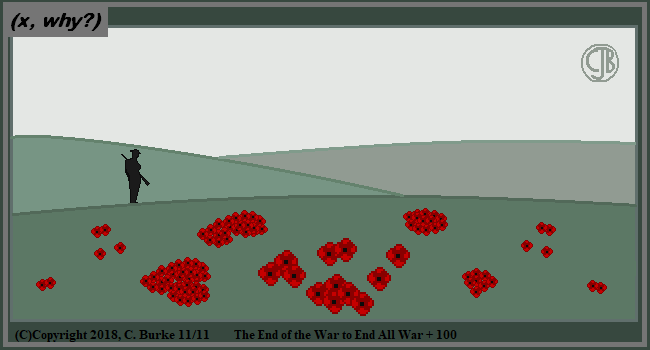Ten years ago, I wondered ten years ago on 11-11-08, just how big a remembrance there would be this year, on the 100th anniversary.

For anyone counting, there are 100 poppies on that field. I originally started placing them in a Fibonacci spiral, but then abandoned that idea.

To all the veterans out there, Thank You.

Come back often for more funny math and geeky comics.## Friday, November 09, 2018

### Plan A for Anniversary

(Click on the comic if you can't see the full image.)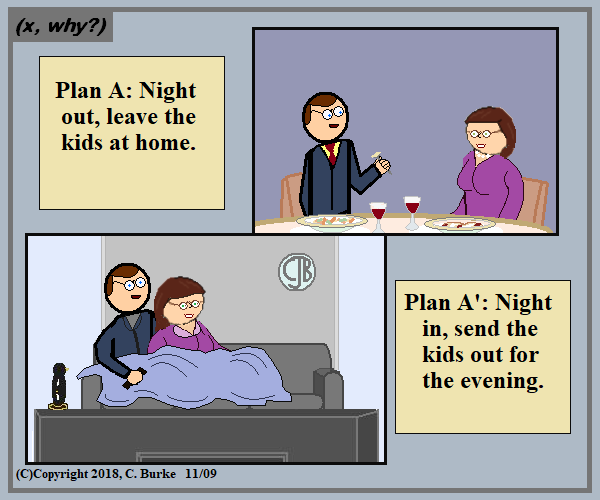It works out that by the time the house is empty on its own, you don't feel the need to leave it to have a good time.

Come back often for more funny math and geeky comics.## Wednesday, November 07, 2018

### Another Hour

(Click on the comic if you can't see the full image.)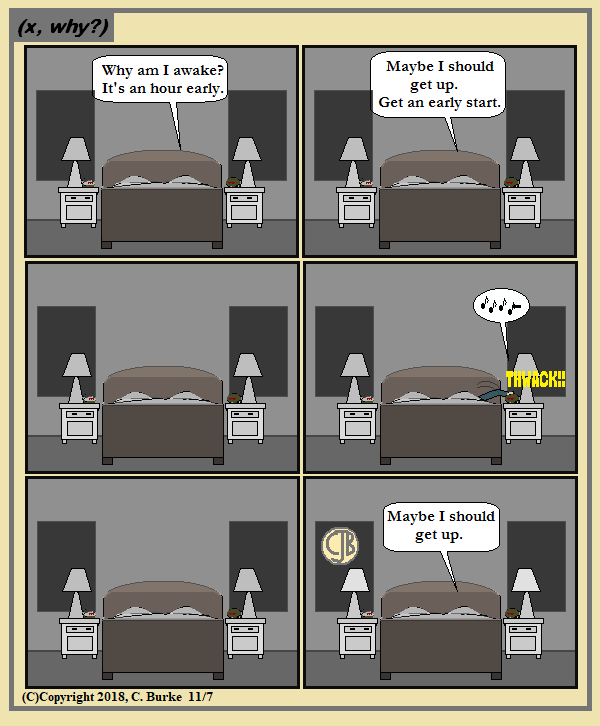Odds are no actual sleep took place within that final hour. Or the snooze afterward.

Come back often for more funny math and geeky comics.## Monday, November 05, 2018

### How Much Candy?

(Click on the comic if you can't see the full image.)